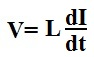﻿ How to Calculate the Voltage of an Inductor

# How to Calculate the Voltage of an InductorTo calculate the voltage across an inductor, the formula is:All you have to know to calculate the voltage across the inductor is L, the Inductance of the Inductor which is expressed in units, Henry, and the derivative of the current going through the inductor.

Example
If the current going through the inductor is 7sin(1000t) and its inductance is 50μH, what is the voltage across the inductor?